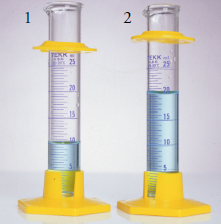# 3.3: Classifying Analytical Techniques

•• Contributed by David Harvey
• Professor (Chemistry and Biochemistry) at DePauw University

Analyzing a sample generates a chemical or physical signal that is proportional to the amount of analyte in the sample. This signal may be anything we can measure, such as mass or absorbance. It is convenient to divide analytical techniques into two general classes depending on whether the signal is proportional to the mass or moles of analyte, or to the analyte’s concentration.

Consider the two graduated cylinders in Figure $$\PageIndex{1}$$, each containing a solution of 0.010 M $$\ce{Cu(NO_3)_2}$$. Cylinder 1 contains 10 mL, or 1.0 × 10-4 moles of $$\ce{Cu^{2+}}$$, and cylinder 2 contains 20 mL, or 2.0 × 10-4 moles of $$\ce{Cu^{2+}}$$. If a technique responds to the absolute amount of analyte in the sample, then the signal due to the analyte, $$S_\ce{A}$$, is

$S_\ce{A} = k_\ce{A} n_\ce{A} \label{3.1}$

where nA is the moles or grams of analyte in the sample, and kA is a proportionality constant. Since cylinder 2 contains twice as many moles of $$\ce{Cu^{2+}}$$ as cylinder 1, analyzing the contents of cylinder 2 gives a signal that is twice that of cylinder 1.

A second class of analytical techniques are those that respond to the analyte’s concentration, CA

$S_\ce{A} = k_\ce{A}C_\ce{A} \label{3.2}$

Since the solutions in both cylinders have the same concentration of $$\ce{Cu^{2+}}$$, their analysis yields identical signals.Figure $$\PageIndex{1}$$: Graduated cylinders containing 0.10 M $$\ce{Cu(NO_3)_2}$$. Although the cylinders contain the same concentration of $$\ce{Cu^{2+}}$$, the cylinder on the left contains 1.0 × 10-4 mol $$\ce{Cu^{2+}}$$ and the cylinder on the right contains 2.0 × 10-4 mol $$\ce{Cu^{2+}}$$.

A technique responding to the absolute amount of analyte is a total analysis technique. Mass and volume are the most common signals for a total analysis technique, and the corresponding techniques are gravimetry and titrimetry. With a few exceptions, the signal for a total analysis technique is the result of one or more chemical reactions involving the analyte. These reactions may involve any combination of precipitation, acid–base, complexation, or redox chemistry. The stoichiometry of the reactions determines the value of kA in Equation $$\ref{3.1}$$. Historically, most early analytical methods used a total analysis technique. For this reason, total analysis techniques are often called “classical” techniques.

Spectroscopy and electrochemistry, in which an optical or electrical signal is proportional to the relative amount of analyte in a sample, are examples of concentration techniques. The relationship between the signal and the analyte’s concentration is a theoretical function that depends on experimental conditions and the instrumentation used to measure the signal. For this reason the value of kA in Equation $$\ref{3.2}$$ must be determined experimentally. Since most concentration techniques rely on measuring an optical or electrical signal, they also are known as “instrumental” techniques.